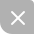# BM算法

RK算法
KMP算法

BM算法思想：有模式串中不存在的字符，那么肯定不匹配，往后多移动几位，提高效率。BM算法定义了两个规则，好后缀规则坏字符规则，同时其匹配的顺序是按照模式串下标从大到小的顺序，即从后往前

#### 坏字符规则• 注意：如果坏字符在模式串中多次出现，取最靠”右“的，因为这样不会让模式串滑动过多，导致本来可能匹配的情况被滑动略过。

void generateBadChar(string p,map<char,int> & badchar){
int n = p.size();
for (int i = 0; i < n; i++){
//如果不再哈希表中，则说明是第一次出现
}else{
//如果出现在哈希表中，说明已经出现了，但是我们存储的是最靠右的哪个位置，因此更新
}
}
}//只根据坏字符规则进行的BM匹配
/*
@param s : 主串
@param p : 模式串
*/
int lens = s.size();
int lenp = p.size();
//i：表示主串与模式串对齐的第一个字符
int i = 0,j;
//i时第i+1此匹配结束的位置
while (i < lens - lenp + 1){
//从后往前的顺序匹配
for(j = lenp - 1; j >= 0; j--){
//匹配失败，直接退出循环，开始滑动模式串
if (s[i+j] != p[j]){
break;
}
}
// j < 0匹配成功
if (j < 0){
return i;
}
//坏字符不在模式串中，直接模式串滑动j+1位
i = i + j + 1;;//等价于 i = i + (j - badchar[s[i+j]])，其中 badchar[s[i+j]] 为 -1
}else{
i = i + (j - badchar[s[i+j]]);
}
}
return -1;
}


#### 好后缀规则1. 在模式串中，查找跟好后缀匹配的另一个子串；
2. 找出好后缀的所有后缀子串
3. 找出模式串的所有前缀子串
4. 找到好后缀中最长的能和模式串的前缀子串匹配的后缀子串//计算suffix和prefix数组
/*
@param p : 模式串
*/
void generateGS(string p,vector<int> &suffix,vector<bool> & prefix){
//suffix[k] = int(i)：表示好后缀的长度为k的好后缀子串，在除了此位置外的最右位置i
//prefix[k] = bool : 表示是否存在长度为k的好后缀子串也是模式串的前缀字串
int n = p.size();
//k：后缀子串的长度
int i,j,k;
for (int i = 0; i < n-1; i++){
j = i;
k = 0;//从j位置开始寻找长度为k的公共后缀字串（每个位置都是从0开始寻找）
while (j >= 0 && p[j] == p[n-1-k]){
--j;
++k;//公共后缀子串长度(模式串尾部取k个出来，分别比较)
suffix[k] = j+1;
//相同后缀子串长度为k时，该子串在b[0,i]中的起始下标
// (如果有多个相同长度的子串，被赋值覆盖，存靠右的)
}
//查找到模式串的头部了
if (j == -1){
prefix[k] = true;//如果公共后缀子串也是模式串的前缀子串
}
}
}//根据坏字符位置j,suffix和prefix，求取移动的位数
/*
@param j : 坏字符位置
@param return : 模式串应该后移位数
*/
int moveByGS(int j,int lenp,vector<int> &suffix,vector<bool> &prefix){
int k = lenp - j - 1;//好后缀长度
//如果模式串中有好后缀，则直接计算后移位数
if (suffix[k] != -1){
return j - suffix[k] + 1;
}
//好后缀是[j+1，m-1], 好后缀的子串肯定小于好后缀，就不能从j+1开始,从j+2开始
for(int r = j + 2; r < lenp; ++r){
//lenp-r是好后缀的子串的长度，如果这个好后缀的子串是模式串的前缀子串
if(prefix[lenp-r] == true)//lenp - r 等价于 m-1-r+1 = m-r = lenp-r
//在上面没有找到相同的好后缀下，移动r位，对齐前缀到好后缀
return r;
}
return lenp;//case3,都没有匹配的，移动lenp位（模式串长度）
}


### 如何选择使用哪种规则

• 分别计算好后缀和坏字符规则往后滑动的位数，取大的，作为滑动位数（还可以避免负数,即反向滑动）
int BMMatching(string s,string p){
int lens = s.size();
int lenp = p.size();
if (lens == 0 || lenp == 0){//存在空串，匹配失败
return -1;
}
vector<int> suffix(lenp,-1);
vector<bool> prefix(lenp,false);
//计算suffix和prefix
generateGS(p,suffix,prefix);
//movelen1：根据坏字符规则求得的滑动位数
//movelen2：根据好后最规则求得的滑动位数
int i = 0,movelen1 = 0,movelen2 = 0,j;
while (i < lens-lenp+1){
for (j = lenp - 1; j >= 0; j--){
if (s[i+j] != p[j]){//遇到坏字符
break;
}
}
if (j < 0){//匹配成功
return i;
}
//坏字符不在模式串中，直接模式串滑动j+1位
movelen1 =  j + 1;;
}else{
}
movelen2 = 0;
if (j < lenp-1){//如果有好后缀
movelen2 = moveByGS(j,lenp,suffix,prefix);
}
//选取滑动位数多的那一个
i = i + max(movelen1,movelen2);
}
return -1;
}


### 性能分析UP更新不错过~
04-10
11-12
03-06283
01-191010
01-179527
06-223万+
03-27865
09-07287
08-11167
04-261874
06-05430
10-101357
11-21112
10-191766
04-19768
09-13529
07-192927
05-2762
03-21981
03-198907

### “相关推荐”对你有帮助么？

•非常没帮助
•没帮助
•一般
•有帮助
•非常有帮助被折叠的  条评论 为什么被折叠?到【灌水乐园】发言Small_Fish25

¥2 ¥4 ¥6 ¥10 ¥20余额支付 (余额：-- )扫码支付获取中扫码支付点击重新获取扫码支付1.余额是钱包充值的虚拟货币，按照1:1的比例进行支付金额的抵扣。
2.余额无法直接购买下载，可以购买VIP、C币套餐、付费专栏及课程。余额充值